# ICTP Portal

##### Sections
« December 2023 »
Su Mo Tu We Th Fr Sa
1 2
3 4 5 6 7 8 9
10 11 12 13 14 15 16
17 18 19 20 21 22 23
24 25 26 27 28 29 30
31

You are here: Home basic_ostream

# basic_ostream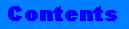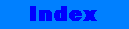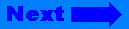## basic_ostream

`basic_ostream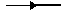basic_iosios_base`

### Data Type and Member Function Indexes(exclusive of constructors and destructors)

 Data Types char_type int_type ios_type off_type ostream ostream_type pos_type traits_type wostream
 Member Functions bool() endl() ends() flush() operator<<() put() seekp() sentry() tellp() write() ~sentry()

### Synopsis

```#include <ostream>
template<class charT, class traits = char_traits<charT> >
class basic_ostream
: virtual public basic_ios<charT, traits>```

### Description

The class basic_ostream defines a number of member function signatures that assist in formatting and writing output to sequences controlled by a stream buffer.

Two groups of member function signatures share common properties: the formatted output functions (or insertors) and the unformatted output functions. Both groups of functions insert characters by calling basic_streambuf member functions. They both begin by constructing an object of class basic_ostream::sentry and, if this object is in good state after construction, the function tries to perform the requested output. The sentry object performs exception-safe initialization, such as controlling the status of the stream or locking it in multithread environment.

Some formatted output functions generate the requested output by converting a value from some scalar to text form and inserting the converted text in the output sequence. The conversion behavior is locale dependent, and directly depend on the locale object imbued in the stream.

### Interface

```template<class charT, class traits = char_traits<charT> >
class basic_ostream
:virtual public basic_ios<charT, traits> {

public:

typedef basic_ostream<charT, traits>   ostream_type;
typedef basic_ios<charT, traits>       ios_type;

typedef traits                         traits_type;
typedef charT                          char_type;

typedef typename traits::int_type      int_type;
typedef typename traits::pos_type      pos_type;
typedef typename traits::off_type      off_type;

explicit basic_ostream(basic_streambuf<charT, traits> *sb);
virtual ~basic_ostream();

class sentry {

public:

explicit sentry(basic_ostream<charT,traits>&);
~sentry();
operator bool ();

};

ostream_type& operator<<(ostream_type& (*pf)(ostream_type&));
ostream_type& operator<<(ios_base& (*pf)(ios_base&));
ostream_type& operator<<(ios_type& (*pf)(ios_type&));

ostream_type& operator<<(bool n);
ostream_type& operator<<(short n);
ostream_type& operator<<(unsigned short n);
ostream_type& operator<<(int n);
ostream_type& operator<<(unsigned int n);
ostream_type& operator<<(long n);
ostream_type& operator<<(unsigned long n);
ostream_type& operator<<(float f);
ostream_type& operator<<(double f);
ostream_type& operator<<(long double f);

ostream_type& operator<<(void *p);

ostream_type& operator<<(basic_streambuf<char_type, traits>& sb);
ostream_type& operator<<(basic_streambuf<char_type, traits> *sb);

ostream_type& put(char_type c);

ostream_type& write(const char_type *s, streamsize n);

ostream_type& flush();

ostream_type& seekp(pos_type );
ostream_type& seekp(off_type , ios_base::seekdir );
pos_type tellp();

protected:

basic_ostream();

};

//global functions

template <class charT, class traits>
basic_ostream<charT,traits>&
operator<<(basic_ostream<charT,traits>&, charT);

template <class charT, class traits>
basic_ostream<charT,traits>&
operator<<(basic_ostream<charT,traits>&, char);

template <class traits>
basic_ostream<char,traits>&
operator<<(basic_ostream<char,traits>&, char);

template <class charT, class traits>
basic_ostream<charT,traits>&
operator<<(basic_ostream<charT,traits>&, const charT*);

template <class charT, class traits>
basic_ostream<charT,traits>&
operator<<(basic_ostream<charT,traits>&, const char*);

template <class traits>
basic_ostream<char,traits>&
operator<<(basic_ostream<char,traits>&, const char*);

template <class traits>
basic_ostream<char,traits>&
operator<<(basic_ostream<char,traits>&, unsigned char);

template <class traits>
basic_ostream<char,traits>&
operator<<(basic_ostream<char,traits>&, signed char);

template <class traits>
basic_ostream<char,traits>&
operator<<(basic_ostream<char,traits>&, const unsigned char*);

template <class traits>
basic_ostream<char,traits>&
operator<<(basic_ostream<char,traits>&, const signed char*);

template<class charT, class traits>
basic_ostream<charT, traits>&
endl(basic_ostream<charT, traits>& os);

template<class charT, class traits>
basic_ostream<charT, traits>&
ends(basic_ostream<charT, traits>& os);

template<class charT, class traits>
basic_ostream<charT, traits>&
flush(basic_ostream<charT, traits>& os);```

### Types

`char_type`

The type char_type is a synonym for the template parameter charT.

`int_type`

The type int_type is a synonym of type traits::in_type.

`ios_type`

The type ios_type is a synonym for basic_ios<charT, traits>.

`off_type`

The type off_type is a synonym of type traits::off_type.

`ostream`

The type ostream is an instantiation of class basic_ostream on type char:

typedef basic_ostream<char> ostream;

`ostream_type`

The type ostream_type is a synonym for basic_ostream<charT, traits>.

`pos_type`

The type pos_type is a synonym of type traits::pos_type.

`traits_type`

The type traits_type is a synonym for the template parameter traits.

`wostream`

The type wostream is an instantiation of class basic_ostream on type wchar_t:

typedef basic_ostream<wchar_t> wostream;

### Constructor

`explicit basic_ostream(basic_streambuf<charT, traits>* sb);`

Constructs an object of class basic_ostream, assigning initial values to the base class by calling basic_ios<charT,traits>::init(sb).

### Destructor

`virtual ~basic_ostream();`

Destroys an object of class basic_ostream.

### Sentry Class

`explicit sentry(basic_ostream<charT,traits>&);`

Prepares for formatted or unformatted output. First if the basic_ios member function tie() is not a null pointer, the function synchronizes the output sequence with any associated stream. If after any preparation is completed the basic_ios member function good() is true, the sentry conversion function operator bool () will return true. Otherwise it will return false. In multithread environment the sentry object constructor is responsible for locking the stream and the stream buffer associated with the stream.

`~sentry();`

Destroys an object of class sentry. If the ios_base member function flags() & unitbuf == true, then flush the buffer. In multithread environment the sentry object destructor is responsible for unlocking the stream and the stream buffer associated with the stream.

`operator bool();`

If after any preparation is completed the ios_base member function good() is true, the sentry conversion function operator bool() will return true else it will return false.

### Insertors

```ostream_type&
operator<<(ostream_type& (*pf) (ostream_type&));```

Calls pf(*this), then return *this. See, for example, the function signature endl(basic_ostream&).

```ostream_type&
operator<<(ios_type& (*pf) (ios_type&));```

Calls pf(*this), then return *this.

```ostream_type&
operator<<(ios_base& (*pf) (ios_base&));```

Calls pf(*this), then return *this. See, for example, the function signature dec(ios_base&).

```ostream_type&
operator<<(bool n);```

Converts the boolean value n, and outputs it into the basic_ostream object's buffer. If the ios_base member function flag() & ios_base::boolalpha is false it tries to write an integer value, which must be 0 or 1. If the boolalpha flag is true, it writes characters according to the locale function numpunct<>::truename() or numpunct<>::falsename().

```ostream_type&
operator<<(short n);```

Converts the signed short integer n, and output it into the stream buffer, then return *this.

```ostream_type&
operator<<(unsigned short n);```

Converts the unsigned short integer n, and output it into the stream buffer, then return *this.

```ostream_type&
operator<<(int n);```

Converts the signed integer n, and output it into the stream buffer, then return *this.

```ostream_type&
operator<<(unsigned int n);```

Converts the unsigned integer n, and output it into the stream buffer, then return *this.

```ostream_type&
operator<<(long n);```

Converts the signed long integer n, and output it into the stream buffer, then return *this.

```ostream_type&
operator<<(unsigned long n);```

Converts the unsigned long integer n, and output it into the stream buffer, then return *this.

```ostream_type&
operator<<(float f);```

Converts the float f and output it into the stream buffer, then return *this.

```ostream_type&
operator<<(double f);```

Converts the double f and output it into the stream buffer, then return *this.

```ostream_type&
operator<<(long double f);```

Converts the long double f and output it into the stream buffer, then return *this.

```ostream_type&
operator<<(void *p);```

Converts the pointer p, and output it into the stream buffer, then return *this.

```ostream_type&
operator<<(basic_streambuf<charT,traits> *sb);```

If sb is null calls the basic_ios member function setstate(badbit). Otherwise gets characters from sb and inserts them into the stream buffer until any of the following occurs:

• end-of-file occurs on the input sequence.

• inserting in the output sequence fails

• an exception occurs while getting a character from sb

If the function inserts no characters or if it stopped because an exception was thrown while extracting a character, it calls the basic_ios member function setstate(failbit). If an exception was thrown while extracting a character it is rethrown.

```ostream_type&
operator<<(basic_streambuf<charT,traits>& sb);```

Gets characters from sb and inserts them into the stream buffer until any of the following occurs:

• end-of-file occurs on the input sequence.

• inserting in the output sequence fails

• an exception occurs while getting a character from sb

If the function inserts no characters or if it stopped because an exception was thrown while extracting a character, it calls the basic_ios member function setstate(failbit). If an exception was thrown while extracting a character it is rethrown.

### Unformatted Functions

```ostream_type&
flush();```

If rdbuf() is not a null pointer, calls rdbuf()->pubsync() and returns *this. If that function returns -1 calls setstate(badbit).

```ostream_type&
put(char_type c);```

Inserts the character c. If the operation fails, calls the basic_ios member function setstate(badbit).

```ostream_type&
seekp(pos_type pos);```

If the basic_ios member function fail() returns false, executes rdbuf()->pubseekpos(pos), which will position the current pointer of the output sequence at the position designated by pos.

```ostream_type&
seekp(off_type off, ios_base::seekdir dir);```

If the basic_ios member function fail() returns false, executes rdbuf()->pubseekpos(off,dir), which will position the current pointer of the output sequence at the position designated by off and dir.

```pos_type
tellp();```

If the basic_ios member function fail() returns true, tellp() returns pos_type(off_type(-1)) to indicate failure. Otherwise it returns the current position of the output sequence by calling rdbuf()-> pubseekoff(0,cur, out).

```ostream_type&
write(const char_type* s, streamsize n);```

Obtains characters to insert from successive locations of an array whose first element is designated by s. Characters are inserted until either of the following occurs:

• n characters are inserted

• inserting in the output sequence fails

In the second case the function calls the basic_ios member function setstate(badbit). The function returns *this.

### Non Member Functions

```template<class charT, class traits>
basic_ostream<charT, traits>&
endl(basic_ostream<charT, traits>& os);```

Outputs a newline character and flush the buffer, then returns os.

```template<class charT, class traits>
basic_ostream<charT, traits>&
ends(basic_ostream<charT, traits>& os);```

Inserts a null character into the output sequence, then returns os.

```template<class charT, class traits>
basic_ostream<charT, traits>&
flush(basic_ostream<charT, traits>& os);```

Flushes the buffer, then returns os.

```template<class charT, class traits>
basic_ostream<charT, traits>&
operator<<(basic_ostream<charT, traits>& os, charT c);```

Outputs the character c of type charT into the basic_ostream object's buffer. Both the stream and the stream buffer are instantiated on type charT. Padding is not ignored.

```template<class charT, class traits>
basic_ostream<charT, traits>&
operator<<(basic_ostream<charT, traits>& os, char c);```

Outputs the character c of type char into the basic_ostream object's buffer. Both the stream and the stream buffer are instantiated on type charT. Conversion from characters of type char to characters of type charT is performed by the basic_ios member function widen. padding is not ignored.

```template<class traits>
basic_ostream<char, traits>&
operator<<(basic_ostream<char, traits>& os, char c);```

Outputs the character c of type char into the basic_ostream object's buffer. Both the stream and the stream buffer are instantiated on type char. Padding is not ignored.

```template<class charT, class traits>
basic_ostream<charT, traits>&
operator<<(basic_ostream<charT, traits>& os, const charT* s);```

Outputs the null-terminated-byte-string s of type charT* into the basic_ostream object's buffer. Both the stream and the stream buffer are instantiated on type charT.

```template<class charT, class traits>
basic_ostream<charT, traits>&
operator<<(basic_ostream<charT, traits>& os, const char* s);```

Outputs the null-terminated-byte-string s of type char* into the basic_ostream object's buffer. Both the stream and the stream buffer are instantiated on type charT. Conversion from characters of type char to characters of type charT is performed by the basic_ios member function widen.

```template<class traits>
basic_ostream<char, traits>&
operator<<(basic_ostream<char, traits>& os, const char* s);```

Outputs the null-terminated-byte-string s of type char* into the basic_ostream object's buffer. Both the stream and the stream buffer are instantiated on type char.

```template<class traits>
basic_ostream<char, traits>&
operator<<(basic_ostream<char, traits>& os, unsigned char c);```

Returns os << (char)c.

```template<class traits>
basic_ostream<char, traits>&
operator<<(basic_ostream<char, traits>& os, signed char c);```

Returns os << (char)c.

```template<class traits>
basic_ostream<char, traits>&
operator<<(basic_ostream<char, traits>& os, unsigned char* c);```

Returns os << (char*)c.

```template<class traits>
basic_ostream<char, traits>&
operator<<(basic_ostream<char, traits>& os, signed char* c);```

Returns os << (char*)c.

### Formatting

The formatting is done through member functions or manipulators.

 Manipulators Member Functions showpos setf(ios_base::showpos) noshowpos unsetf(ios_base::showpos) showbase setf(ios_base::showbase) noshowbase unsetf(ios_base::showbase) uppercase setf(ios_base::uppercase) nouppercase unsetf(ios_base::uppercase) showpoint setf(ios_base::showpoint) noshowpoint unsetf(ios_base::showpoint) boolalpha setf(ios_base::boolalpha) noboolalpha unsetf(ios_base::boolalpha) unitbuf setf(ios_base::unitbuf) nounitbuf unsetf(ios_base::unitbuf) internal setf(ios_base::internal, ios_base::adjustfield) left setf(ios_base::left, ios_base::adjustfield) right setf(ios_base::right, ios_base::adjustfield) dec setf(ios_base::dec, ios_base::basefield) hex setf(ios_base::hex, ios_base::basefield) oct setf(ios_base::oct, ios_base::basefield) fixed setf(ios_base::fixed, ios_base::floatfield) scientific setf(ios_base::scientific, ios_base::floatfield) resetiosflags (ios_base::fmtflags flag) setf(0,flag) setiosflags (ios_base::fmtflags flag) setf(flag) setbase(int base) see above setfill(char_type c) fill(c) setprecision(int n) precision(n) setw(int n) width(n)

### Description

 showpos Generates a + sign in non-negative generated numeric output showbase Generates a prefix indicating the numeric base of generated integer output uppercase Replaces certain lowercase letters with their uppercase equivalents in generated output showpoint Generates a decimal-point character unconditionally in generated floating-point output boolalpha Insert and extract bool type in alphabetic format unitbuf Flushes output after each output operation internal Adds fill characters at a designated internal point in certain generated output, or identical to right if no such point is designated left Adds fill characters on the right (final positions) of certain generated output right Adds fill characters on the left (initial positions) of certain generated output dec Converts integer input or generates integer output in decimal base hex Converts integer input or generates integer output in hexadecimal base oct Converts integer input or generates integer output in octal base fixed Generates floating-point output in fixed-point notation scientific Generates floating-point output in scientific notation resetiosflagss (ios_base::fmtflags flag) Resets the fmtflags field flag setiosflags (ios_base::fmtflags flag) Set up the flag flag setbase(int base) Converts integer input or generates integer output in base base. The parameter base can be 8, 10 or 16. Setfill(char_type c) Set the character used to pad (fill) an output conversion to the specified field width setprecision(int n) Set the precision (number of digits after the decimal point) to generate on certain output conversions setw(int n) Set the field with (number of characters) to generate on certain output conversions

### Examples

```//
// stdlib/examples/manual/ostream1.cpp
//
#include<iostream>
#include<ostream>
#include<sstream>
#include<iomanip>

void main ( )
{
using namespace std;

float f= 3.14159;
int   i= 22;
char* s= "Randy is the king of stdlib";

// create a read/write stringbuf object on tiny char
// and attach it to an istringstream object
istringstream in( ios_base::in | ios_base::out );

// tie the ostream object to the istringstream object
ostream out(in.rdbuf());

out << "test beginning !" << endl;

out << hex << i <<endl;

// set the field width to 10
// set the padding character to '@'
// and output i in octal
out << setw(10) << oct << setfill('@') << i << endl;

// set the precision to 2 digits after the separator
// output f
out << setprecision(3) << f << endl;

// output the 17 first characters of s
out.write(s,17);

// output a newline character
out.put('\n');

// output s
out << s << endl;

// output the all buffer to standard output
cout << in.rdbuf();
}

//
// stdlib/examples/manual/ostream2.cpp
//
#include<iostream>
#include<ostream>
#include<sstream>

void main ( )
{
using namespace std;

float f= 3.14159;
wchar_t* s= L"Kenavo !";

// create a read/write stringbuf object on wide char
// and attach it to an wistringstream object
wistringstream in( ios_base::in | ios_base::out );

// tie the wostream object to the wistringstream object
wostream out(in.rdbuf());

out << L"test beginning !" << endl;

// output f in scientific format
out << scientific << f <<endl;

// store the current put-pointer position
wostream::pos_type pos = out.tellp();

// output s
out << s << endl;

// output the all buffer to standard output
wcout << in.rdbuf() << endl;

// position the get-pointer
in.seekg(pos);

// output s
wcout << in.rdbuf() << endl;
}
```

char_traits(3C++), ios_base(3C++), basic_ios(3C++), basic_streambuf(3C++), basic_iostream(3C++)

Working Paper for Draft Proposed International Standard for Information Systems--Programming Language C++, Section 27.6.2.1

### Standards Conformance

ANSI X3J16/ISO WG21 Joint C++ Committee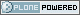This site conforms to the following standards: